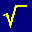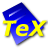# EqualX LaTeX Equation Editor

0.7.1

EqualX LaTeX Equation Editor is a program that will assists you while you type your LaTeX equations. The Templates Toolbar provides common equations to choose from. Just start typing and a completion menu appears with symbols to choose. It gives you the power of TeX/LaTeX for writing equations.

Open source
OS:
Windows 7/10/8.1/8/Vista/XP
Date:
File size:
4.15 MB
Category rank:
812
All software rank:
19 253

## More about EqualX LaTeX Equation Editor

You can download the 0.7.1 version of this program 4.15 MB from this source, and you can also find this program as EqualX. Mihai Niculescu distributes the software free of charge. This tool belongs to Office Software in the Productivity category. Users from all over the world install the versions 0.7 and 0.5 on Windows 7. The most popular installation file name for EqualX LaTeX Equation Editor is equalx.exe. The antivirus scan of the download link proved it to be safe.Download
EqualX LaTeX Equation Editor 0.7.1

## Comments on EqualX LaTeX Equation Editor

Thank you for rating the program!

### Useful softwareMathML .NET Control
Equation editor component for the .NET Framework.MathFlow for XMetaL
MathFlow Editor is a native MathML equation editor that works with XMetaL.MathType
Edit mathematical equations for Word files, web pages, and LaTeX documents.WinShell
Free multilingual (IDE) for LaTeX and TeX.IguanaTex
PowerPoint plug-in which allows you to insert LaTeX equations.